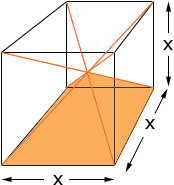SEARCH HOMEMath Central Quandaries & QueriesFrom Becky: why is the formula for finding the volume of a pyramid divide by 3?Hi Becky,

The volume of a pyramid is 1/3 × (the area o the base) × (the height). I don't know an intuitive way to demonstate why the fraction 1/3 appears. It follows from a much more general result that Bruce mentioned at the bottom to his response to an earlier question. I can show you in one particular case why the 1/3 appears.

If the pyramid has a square base with side length x and the height of the pryamid is x/2 then you can put 6 of these pyramids together to form a cube as in the diagram below.The volume of the cube is x3 and hence the volume of the pyramid is 1/6 x3. But

1/6 x3 = 1/3 × x2 × (1/2 x) = 1/3 × (the area of the base) × (the height).

Penny* Registered trade mark of Imperial Oil Limited. Used under license.Math Central is supported by the University of Regina and the Imperial Oil Foundation.